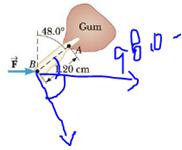Chapter 8, Problem 6P

Chapter
Section
Textbook Problem

A dental bracket exerts a horizontal force of 80.0 N on a tooth at point B in Figure P8.6. What is the torque on the root of the tooth about point A?To determine
The torque on the root of the tooth about point A .

Explanation
For torque on the beam about the point A , the perpendicular component of force on the beam is multiplied with their corresponding location.

Given info: The length of the root of the teeth is 1.20cm , the force on the root is 80.0N , and the orientation of the root from vertical line is 48.0° .

The formula for the net torque on the beam is,

τ=rFcosθ

• r is length of the root.
• F is the force on the tooth due to dental bracket.
• b is length of the beam.
• θ is the angle of the force.

Substitute 80.0N for F , 48.0° for θ , and 1.20cm for r to find τ

Still sussing out bartleby?

Check out a sample textbook solution.

See a sample solution

The Solution to Your Study Problems

Bartleby provides explanations to thousands of textbook problems written by our experts, many with advanced degrees!

Get Started

Which of the following is an example of a processed food? a. carrots b. bread c. nuts d. watermelon

Nutrition: Concepts and Controversies - Standalone book (MindTap Course List)

Is cis-trans isomerism possible in alkanes?

Introduction to General, Organic and Biochemistry

How does the Bobcock model explain the sunspot cycle?

Horizons: Exploring the Universe (MindTap Course List)